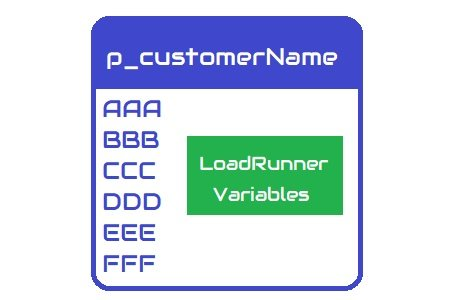There is always a confusion related to variables in LoadRunner script. Because some variables require defining at the start of the script while some variables can be used directly without any initialization. Let’s discuss what is the exact difference in them?

Basically, there are two types of variable in the LoadRunner:

## 1. Language-Specific Variables:

Language-specific variables are those variables which need both declaration and definition (optional) at the start of the script such as:

``````int count;

float amount;``````

You can use these variables to perform some mathematical or file handling operations in the script.

These variables do not require any explicit declaration. When a function calls then LoadRunner declares these variables internally. Such as:

``````lr_save_string("ABC","myVar");

web_reg_save_param("correlationVar", "LB =", "RB =", LAST);``````

LoadRunner variable is used with curly braces in the script. For example, the correlation variable will be written as {correlationVar}.

## Conversion:

### From language-specific variable to LoadRunner variable:

As mentioned above to copy the value of language-specific variable to LoadRunner variable, lr_save_int() and lr_save_string() functions are used.

Example:

``````int x = 5;
..
lr_save_int(x, "lrVarInt");``````

Here, x is a language-specific variable and lrVarInt is a LoadRunner variable. lr_save_int function copies the value of the language-specific variable in LoadRunner variable. Now the lrVarInt has value 5 and can be used like {lrVarInt} in the script.

Similarly, you can use lr_save_string() function to copy the value of string variables into LoadRunner variables.

### From LoadRunner variable to Language-Specific variable:

lr_eval_string() is an important function in LoadRunner which helps to convert LoadRunner variable into language-specific variable. Whenever you want to use the value of LoadRunner variable in a language-specific function then you need to first evaluate the parameter. lr_eval_string function evaluates the parameter and returns the value in a string data type.

Example:

A LoadRunner variable (say lrVarStr) stores a value ‘PerfMatrix’, so lr_eval_string(“{lrVarStr}”) will return ‘PerfMatrix’ which will be copied in a language-specific variable (say lsVarStr).

``````char lsVarStr;
..
strcpy(lsVarStr, lr_eval_string("{lrVarStr}");``````

Similarly, let’s assume another LoadRunner variable (say lrVarInt) stores a value ‘100’, so lr_eval_string(“{lrVarInt}”) will return ‘100’ in string format which will be first converted into integer using atoi function and then assigned to a language-specific variable (say lsVarInt).

``````int lsVarInt;
..
lsVarInt = atoi(lr_eval_string("{lrVarStr}"));``````

Note:

1. lr_eval_string() function always returns string value irrespective of datatype of parameter
2. In case of an integer, the value returned by lr_eval_string() needs to be converted from string to integer explicitly
3. atoi() function converts any string to an integer. Hence I used it in the above example.

Using above methods you can use the value of LoadRunner and Language-Specific variables in both ways depends on the requirement.